# Concentric-Ring Skin-Effect Model

The skin effect does not depend on any mysterious underlying forces. It may be completely predicted based on one simple, lumped-element equivalent model called the concentric-ring model.

Table 2.5. Assumptions Made by z BETTER

Conductor Geometry

L i (0)

Round wire of any radius not in close proximity to a return path ( k p = 1)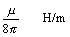Wire of any convex cross section with perimeter p and area aRectangular wire of size w x t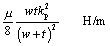Square wire of size w x w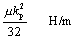Very thin rectangular wire, t << wImagine a round conductor divided lengthwise into concentric tubes, like the growth rings on a tree trunk. In this model the current proceeds absolutely parallel to the wire's central axis. Because current naturally passes straight down the trunk, parallel to the dividing boundaries between the rings, you may insulate the rings from each other without affecting the circuit. You now have a collection of n distinct, isolated conductors, shorted together only at each end of the trunk.

You may now separately consider the inductance of each ring. The inner rings, like long, skinny pipes, have more inductance than the outer rings, which are fatter. You know that at high frequencies, current follows the path of least inductance. Therefore, at high frequencies you should expect more current in the outer tree rings than in the inner. That is exactly what happens. At high frequencies the current crowds into the outermost rings. At low frequencies current partitions itself according to the resistance of each ring, while at high frequencies it partitions itself according of the inductance of each ring.

This simple concentric-ring idea motivates the idea of a redistribution of current at higher frequencies. What it doesn't do, however, is properly indicate the magnitude of the effect. The skin-effect mechanism is far more powerful than just the ratio of individual concentric-ring inductances. Mutual inductance between the rings actually bunches the current much more tightly onto the outer rings than you might at first imagine. The general setup for constructing a tree-ring model, including the mutual inductance, is discussed in the article "Modeling Skin Effect." The concentric-ring circuit model, if taken to an extreme (hundreds of thousands of rings), properly predicts both skin-effect resistance and skin-effect inductance, at low and high frequencies, for a circular conductor.

2.9.1 Modeling Skin Effect

## Article first published in EDN Magazine , April 12, 2001

Why does high-frequency current flow only on the outer surface of a printed-circuit trace?

”Dipak Patel

Magnetic fields cause the behavior you describe. The technical name for this property is the skin effect . It happens in all conductors. If you really like mathematics, the following section will help you to better understand why the skin effect happens. If not, this might be a good time to step out for a cup of tea.

I'll start our discussion with a perfect coaxial cable. Figure 2.18 divides the center conductor of this cable into a series of three concentric rings with radii r , r 1 , and r 2 ( meters ). A lumped-element model of this simple circuit demonstrates that high-frequency signal current flows only on ring number 2.

Figure 2.18. The total magnetic flux within the shaded region equals L 1,0 .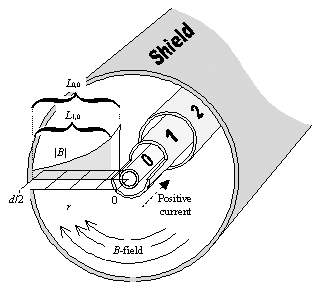At high frequencies magnetic interactions between conductors become significant.

At DC, the longitudinal voltage drop per meter across each conductor n equals the current i n times its resistance per meter. You can express this relation in matrix terms by defining a square matrix R relating the circuit voltages V to the currents I :

Equation 2.60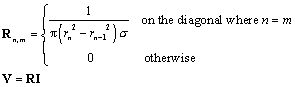where vector V represents the longitudinal voltage across the ends of each conductor (V/m), vector I represents the current in each conductor (A), R is a matrix of resistance values ( W /m), s is the conductivity of the center conductor (S/m), and r n represents the radius of each concentric ring (m), with r = 0.

At high frequencies magnetic interactions between the conductors become significant. Figure 2.18 illustrates the pattern of magnetic fields between the center conductor and shield. The magnetic lines of force ( B -field) form concentric circles around the conductive rings. The drawing plots the field intensity, B , versus radial position, r , assuming a positive signal current of 1A flowing in ring 0 with the return current flowing in the shield. The field strength is zero within the interior of ring 0 and zero outside the shield, and varies with 1/ r (Ampere's law) in between. The exact field intensity for a current of 1A on conductor m is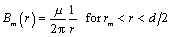, where m is the magnetic permeability of the dielectric material (usually 4 p ·10 “7 H/m).

You calculate the mutual inductance per meter between conductors n and m (for n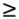m ) using Faraday's law as the integral of the magnetic-field strength, B m , taken over the range from conductor n (at radial position r n ) to the shield (at radial position d /2). Integrating 1/ r yields ln( r ) and the following matrix equations for mutual inductance (values for n < m are found using symmetry: L n,m = L m,n ):

Equation 2.61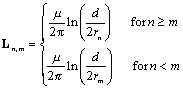The system equation for the whole coaxial circuit sums both resistive and inductive terms as V = ( R + j w L ) I . As an example, the following is the inductance matrix for a three-ring model of an RG-58/U coaxial cable:

Equation 2.62Now comes the main point of this article: The terms in the right-hand column of L are all the same. Why? Because ring 2 concentrates all its flux into the space between ring 2 and the shield. Therefore, all other rings couple 100% to this flux.

The constancy of the right-hand column greatly simplifies the solution to the system equation. To solve this equation, you must find a pattern of currents I such that ( R + j w L ) I generates the same longitudinal voltage across every ring. You need the same voltage across every ring because the rings are all connected together at their ends. If you operate at a frequency so high that the R term becomes insignificant compared to j w L , the solution is simple. Just fill in the last element of I , leaving all others zero. This solution peels off only the right-hand column of L , properly generating the same voltage for every ring. This is one of the few matrix problems you can solve by inspection.

The simple solution says that at high frequencies the signal current flows only on the outer ring as governed by matrix L . At DC, the current distributes itself more evenly, according to matrix R . At middle frequencies, you get a mixture of both effects. That's the nature of the skin effect.

Real-world conductors behave in a similar manner, as if they were made from a continuum of infinitely thin concentric rings. At higher and higher frequencies, the current squeezes more and more tightly against the surface of the conductor, progressively decreasing the useful current-carrying cross section of the conductor and raising its effective resistance.

2.9.2 Regarding Modeling Skin Effect

## Email correspondence received July 17, 2001 SE HO YOU writes

You have shown an inductance matrix and indicated that since all the entries in the right column of the matrix are the same, ring number 2 concentrates all its flux into the space between ring number 2 and the shield.

Would you please explain why flux concentrates around ring number 2? The behavior of the flux comes first, I think. Then, the inductance matrix should be just a mathematical expression of how nature works. Could you explain more physically?

Eddy currents flowing in the outer ring create a magnetic shield through which flux cannot penetrate . The shielding effect of the outermost ring therefore prevents flux from reaching the inner rings. Receiving no electromagnetic impulsion from changing flux, no current flows on the inner rings.

At low frequencies the eddy currents in the outer ring are impeded by the resistance of the copper , so the shielding effect is imperfect. With an imperfect shield, some magnetic fields do reach the interior and some current does indeed flow on the inner rings.

As you go to higher and higher frequencies, however, the shielding effect becomes more pronounced. The improved shielding effect successively robs the inner rings of more and more flux (and current).High-Speed Signal Propagation[c] Advanced Black Magic
ISBN: 013084408X
EAN: N/A
Year: 2005
Pages: 163